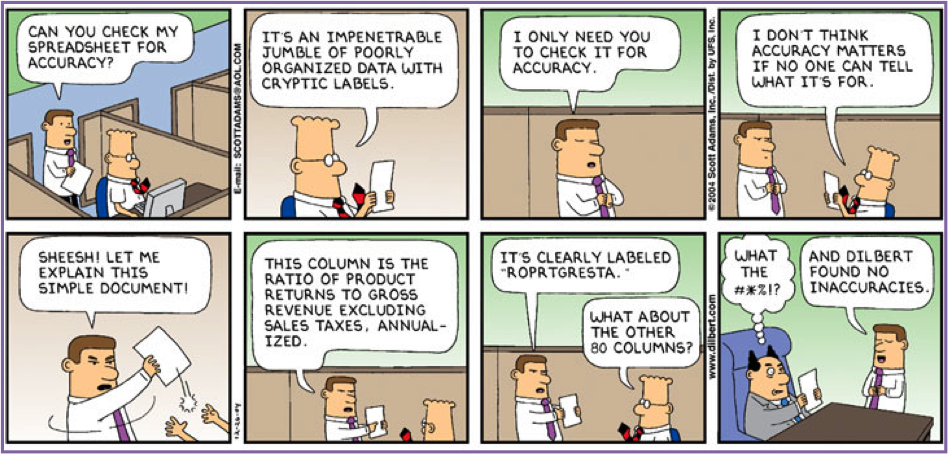D-cide© can be described as a visual spreadsheet or visual model editor. Some of the important features of D-cide© are as follows:

1. Provides an easier and more visual method to build, edit, manipulate and explain a spreadsheet model in a collaborative model-building environment.

2. Allows the user to understand how solutions are generated through using algebraic relationships and natural-language processing instead of formulas constructed behind the scenes in Excel.

3. Interdependencies are visually represented by arrows connecting different objects in a model and showing the logical and mathematical links between them.

4. Offers the ability for the creation of different roles for an element (i.e. Constant, Variable, Self-Reference Variable, and Series).1. Transparency: Traditional spreadsheet programs hide the logic of the model behind columns and rows of data. D-cide© makes it easy to understand how the numbers are generated by using algebraic relationships and natural-language processing instead of cells and formulas.

2. Dependency: Dependencies are hard to see in spreadsheets. The elements of the model in D-cide© are visually represented on a page, and the logical and mathematical links between them are shown by connecting arrows.

3. Role: The cells in a spreadsheet all have identical roles expressed by formulas. There is no distinction among the roles of elements in spreadsheets. In D-cide©, elements have different roles (i.e. Constant, Variable, Self-Reference Variable, and Series).

4. View: Spreadsheets are limited to show either the formulas within the cells or their calculated values at a given time. D-cide© shows the model (input) and the solution (output) side-by-side.

5. Complication:  When working with large spreadsheets, it is difficult to follow the logic of the model by looking up the formulas in the cells.  Editing and refining the model becomes a difficult task.

6. Portability: D-cide© supports Dynamic Data Exchange (DDE). The results can be exported to Excel for further manipulation or graphing.